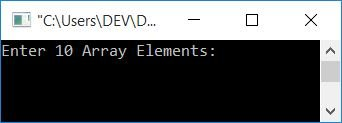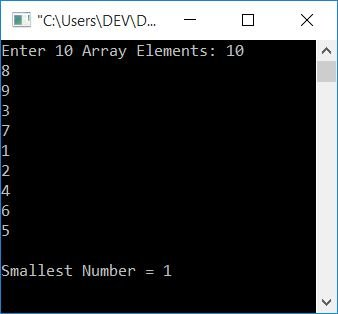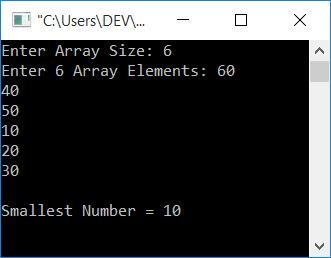# C program to find the smallest number in an array

In this article, you will learn and get code for finding the smallest number in an array with and without using a user-defined function.

To find the smallest element in a given array in C programming, you must first ask the user to enter all of the array's elements. Then, as shown in the program below, find smalls from all:

```#include<stdio.h>
#include<conio.h>
int main()
{
int arr, small, i;
printf("Enter 10 Array Elements: ");
for(i=0; i<10; i++)
scanf("%d", &arr[i]);
i=0;
small=arr[i];
while(i<10)
{
if(small>arr[i])
small = arr[i];
i++;
}
printf("\nSmallest Number = %d", small);
getch();
return 0;
}```

This program was built and runs under the Code::Blocks IDE. Here is its sample run:Now supply any 10 numbers as array elements, say 10, 8, 9, 3, 7, 1, 2, 4, 6, 5, and the ENTER key to see the following output:After receiving the input, which is 10 numbers from the user, as array elements, The value at index 0 (the first number) gets initialized to a "small" variable (assuming that the first number is the smallest one). Then it compares it with the rest of the nine numbers one by one using a while loop and checks whether it is greater or not. If it is, simply set that value to "small" and continue to check for value at the next index until all ten numbers have been compared.Finally, print the value of "small" as output.

## Find the smallest element using a user-defined function

Let's create another program that does the same job with an extra feature added to it. The extra feature is that the user can define the size of the array and then enter elements of that size. This program finds the smallest using a user-defined function, findSmallest().

```#include<stdio.h>
#include<conio.h>
int findSmallest(int [], int);
int main()
{
int arr, small, i, size;
printf("Enter Array Size: ");
scanf("%d", &size);
printf("Enter %d Array Elements: ", size);
for(i=0; i<size; i++)
scanf("%d", &arr[i]);
small=findSmallest(arr, size);
printf("\nSmallest Number = %d", small);
getch();
return 0;
}
int findSmallest(int arr[], int n)
{
int i=0, small;
small=arr[i];
while(i<n)
{
if(small>arr[i])
small = arr[i];
i++;
}
return small;
}```

Here is its sample run:#### The same program in different languages

C Quiz

« Previous Program Next Program »